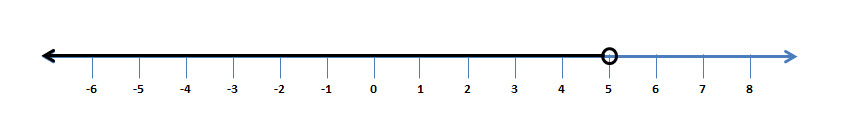If$x$ and$y$ are two quantities such that$x \neq y$; then any of the following conditions could be true:

1.$x > y$
2.$x \geq y$
3.$x < y$
4.$x \leq y$

If$a, b$ and$c$ are real numbers, then each of the following is called a linear inequation in one variable:

1.$ax+b > c$
2.$ax+b
3.$ax+b \geq c$
4.$ax+b \leq c$

As you would have noticed already, the signs$>, <, \geq, \leq$ are called signs of inequality.

Replacement Set and Solution Set in Set Notation

The set from which the value of the variable$x$ is chosen is called Replacement Set and its subsets, whose elements satisfy the inequation are called solution sets.

Let’s take the following example:

If the inequation is$x < 5$, if

1. The replacement set$= N \ i.e \ \{1, 2, 3, 4, 5, 6, ... \}$, then the solution  set$= \{1, 2, 3, 4 \}$
2. The replacement set$= W \ i.e \ \{0, 1, 2, 3, 4,5 ,6 ,7,... \}$, then the solution set$= \{0, 1, 2, 3, 4 \}$

We can also describe the solution set in set builder form  i.e$\{x:x \in N \ and \ x < 5 \}$  or as in the second case$\{x:x \in W \ and \ x < 5 \}$

If the inequation is$x \leq 5$, if

1. The replacement set$= N \ i.e \ \{1, 2, 3, 4, 5, 6, ... \}$, then the solution  set$= \{1, 2, 3, 4, 5 \}$
2. The replacement set$= W \ i.e \ \{0, 1, 2, 3, 4,5 ,6 ,7,... \}$, then the solution set$= \{0, 1, 2, 3, 4, 5 \}$

We can also describe the solution set in set builder form  i.e$\{x:x \in N \ and \ x \leq 5 \}$  or as in the second case$\{x:x \in W \ and \ x \leq 5 \}$

You could also represent the above solutions on a number line as well. Please note the following notations:$\circ$ marks the end of the range with a strict inequality$( < \ or \ > )$$\bullet$ marks the end of the range with a strict inequality$( \leq \ or \ \geq )$

Therefore, for$\{x:x \in I \ and \ x < 5 \}$ we can draw as follows….Therefore for$\{x:x \in I \ and \ x \leq 5 \}$ we can represent as follows….